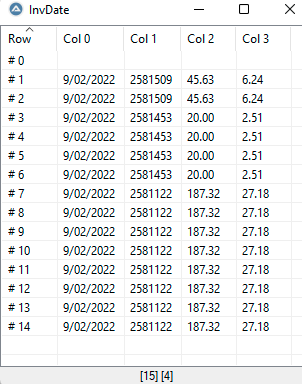# Add values to 2D array in a for loop

## Recommended Posts

I have 4 webpages in an array and I navigate through them using a FOR loop. In each loop (which is a webpage) I need to extract data and add them to an array. So, I've created a 2D array like this: \$dataArray

And using a for loop I wanna insert data to its row and column, but for some reason it doesn't save the data into the 2D array. The data I want to add to the 2D arrays are: \$InvDate, \$InvID, \$MonChar, \$TaxFee.

```Global \$dataarray

\$oIE = _IEAttach("Webpage title")

Local \$oCollection = _IETagNameGetCollection(\$oIE, "label")
Local \$oObj = Null
For \$r=0 to Ubound(\$dataarray,1)
For \$c=0 to Ubound(\$dataarray,2)

For \$oLabel In \$oCollection
If _IEPropertyGet(\$oLabel, "innerText") = "Invoice Date:" Then
\$oObj = \$oLabel.nextSibling.nextSibling
\$InvDate = _IEPropertyGet(\$oObj, "innerText")

EndIf
If _IEPropertyGet(\$oLabel, "innerText") = "Invoice #:" Then
\$oObj = \$oLabel.nextSibling.nextSibling
Global \$InvID = _IEPropertyGet(\$oObj, "innerText")
;MsgBox(0,"Invoice #",_IEPropertyGet(\$oObj, "innerText"))
EndIf
If _IEPropertyGet(\$oLabel, "innerText") = "Monthly Charges:" Then
\$oObj = \$oLabel.nextSibling.nextSibling
Global \$MonChar = _IEPropertyGet(\$oObj, "innerText")
;MsgBox(0,"Monthly Charges",_IEPropertyGet(\$oObj, "innerText"))
EndIf
If _IEPropertyGet(\$oLabel, "innerText") = "Taxes and Fees:" Then
\$oObj = \$oLabel.nextSibling.nextSibling
Global \$TaxFee = _IEPropertyGet(\$oObj, "innerText")
;MsgBox(0,"Taxes and Fees",_IEPropertyGet(\$oObj, "innerText"))
EndIf

Next
;\$dataarray[\$r][\$c+1] = \$InvDate
;MsgBox(0,"Invoice Date",\$InvDate)
ConsoleWrite(\$dataarray &  @TAB)
Next
ConsoleWrite(@CRLF)
Next
Next
_ArrayDisplay(\$dataarray,"InvDate")```

Edited by SaeidN
##### Share on other sites

When using _ArrayAdd, just make sure each item is separated by a piple "|" for example:

```For \$linkarr In \$linkarray
\$oIE = _IEAttach("Webpage title")
Local \$oCollection = _IETagNameGetCollection(\$oIE, "label")
Local \$oObj = Null
For \$r=0 to Ubound(\$dataarray,1)
For \$c=0 to Ubound(\$dataarray,2)
Local \$InvDate = "", \$InvID = "", \$MonChar = "", \$TaxFee = ""
For \$oLabel In \$oCollection
If _IEPropertyGet(\$oLabel, "innerText") = "Invoice Date:" Then
\$oObj = \$oLabel.nextSibling.nextSibling
\$InvDate = _IEPropertyGet(\$oObj, "innerText")
EndIf
If _IEPropertyGet(\$oLabel, "innerText") = "Invoice #:" Then
\$oObj = \$oLabel.nextSibling.nextSibling
\$InvID = _IEPropertyGet(\$oObj, "innerText")
EndIf
If _IEPropertyGet(\$oLabel, "innerText") = "Monthly Charges:" Then
\$oObj = \$oLabel.nextSibling.nextSibling
\$MonChar = _IEPropertyGet(\$oObj, "innerText")
EndIf
If _IEPropertyGet(\$oLabel, "innerText") = "Taxes and Fees:" Then
\$oObj = \$oLabel.nextSibling.nextSibling
\$TaxFee = _IEPropertyGet(\$oObj, "innerText")
EndIf
Next
_ArrayAdd(\$adataarray, \$InvDate & "|" & \$InvID & "|" & \$MonChar & "|" & \$TaxFee)
Next
Next
Next
_ArrayDisplay(\$dataarray,"InvDate")```

Edited by Subz
Added clearing the items
##### Share on other sites

I've change that arrayadd line to this, now it loops more than once, and saves couple values of same. but it should go through it once.

That's my array, this should be 3 rows, I don't know why it's 15Edited by SaeidN
Remove Quote
##### Share on other sites

Since I can't test it's difficult to say, but maybe something like, you may need to adjust as required.

```For \$linkarr In \$linkarray
\$oIE = _IEAttach("Webpage title")
Local \$oCollection = _IETagNameGetCollection(\$oIE, "label")
Local \$oObj = Null
For \$r=0 to Ubound(\$dataarray,1)
For \$c=0 to Ubound(\$dataarray,2)
Local \$InvDate = "", \$InvID = "", \$MonChar = "", \$TaxFee = ""
For \$oLabel In \$oCollection
If _IEPropertyGet(\$oLabel, "innerText") = "Invoice Date:" Then
\$oObj = \$oLabel.nextSibling.nextSibling
\$InvDate = _IEPropertyGet(\$oObj, "innerText")
EndIf
If _IEPropertyGet(\$oLabel, "innerText") = "Invoice #:" Then
\$oObj = \$oLabel.nextSibling.nextSibling
\$InvID = _IEPropertyGet(\$oObj, "innerText")
EndIf
If _IEPropertyGet(\$oLabel, "innerText") = "Monthly Charges:" Then
\$oObj = \$oLabel.nextSibling.nextSibling
\$MonChar = _IEPropertyGet(\$oObj, "innerText")
EndIf
If _IEPropertyGet(\$oLabel, "innerText") = "Taxes and Fees:" Then
\$oObj = \$oLabel.nextSibling.nextSibling
\$TaxFee = _IEPropertyGet(\$oObj, "innerText")
EndIf
Next
Local \$sData = \$InvDate & "|" & \$InvID & "|" & \$MonChar & "|" & \$TaxFee
If Not \$sData = "|||" Then _ArrayAdd(\$adataarray, \$sData)
Next
Next
Next
_ArrayDisplay(\$dataarray,"InvDate")```

##### Share on other sites

It goes through all 4 if loops couple times, instead of one time for each webpage.

Also, this error is stupid.

(95) : ==> Variable used without being declared.:

Local \$sData = \$InvDate & "|" & \$InvID & "|" & \$MonChar & "|" & \$TaxFee
Local \$sData = ^ ERROR

Edited by SaeidN
Remove Quote
##### Share on other sites

As mentioned unable without a URL and fullscript unable to test, but I didn't change anything with the loops or IE functions.

You should add error checking to fix any issues (see below) and maybe you need to add an ExitLoop after _ArrayAdd, but again as I can't test you'll need to work that out.

PS: Please don't include my post when replying as it just makes the thread difficult to read,

```For \$linkarr In \$linkarray
\$oIE = _IEAttach("Webpage title")
Local \$oCollection = _IETagNameGetCollection(\$oIE, "label")
Local \$oObj = Null
For \$r=0 to Ubound(\$dataarray,1)
For \$c=0 to Ubound(\$dataarray,2)
Local \$InvDate = "", \$InvID = "", \$MonChar = "", \$TaxFee = ""
For \$oLabel In \$oCollection
If _IEPropertyGet(\$oLabel, "innerText") = "Invoice Date:" Then
\$oObj = \$oLabel.nextSibling.nextSibling
\$InvDate = _IEPropertyGet(\$oObj, "innerText")
If @error Then \$InvDate = ""
EndIf
If _IEPropertyGet(\$oLabel, "innerText") = "Invoice #:" Then
\$oObj = \$oLabel.nextSibling.nextSibling
\$InvID = _IEPropertyGet(\$oObj, "innerText")
If @error Then \$InvID = ""
EndIf
If _IEPropertyGet(\$oLabel, "innerText") = "Monthly Charges:" Then
\$oObj = \$oLabel.nextSibling.nextSibling
\$MonChar = _IEPropertyGet(\$oObj, "innerText")
If @error Then \$MonChar = ""
EndIf
If _IEPropertyGet(\$oLabel, "innerText") = "Taxes and Fees:" Then
\$oObj = \$oLabel.nextSibling.nextSibling
\$TaxFee = _IEPropertyGet(\$oObj, "innerText")
If @error Then \$TaxFee = ""
EndIf
Next
Local \$sData = \$InvDate & "|" & \$InvID & "|" & \$MonChar & "|" & \$TaxFee
If Not \$sData = "" Then _ArrayAdd(\$adataarray, \$sData)
Next
Next
Next
_ArrayDisplay(\$dataarray,"InvDate")```

## Create an account or sign in to comment

You need to be a member in order to leave a comment

## Create an account

Sign up for a new account in our community. It's easy!

Register a new account

• ### Recently Browsing   0 members

×

• Wiki

• Back

• #### Beta

• Git
• FAQ
×
• Create New...